Disclaimer: This is an example of a student written essay.

Any scientific information contained within this essay should not be treated as fact, this content is to be used for educational purposes only and may contain factual inaccuracies or be out of date.

# Simple Harmonic Motion Experiment

 ✅ Paper Type: Free Essay ✅ Subject: Physics ✅ Wordcount: 1560 words ✅ Published: 1st Feb 2018

• Kisal Jayakody

Technical Report

In this experiment, a motion sensor is used to measure the position of an oscillating mass as a function of time. The frequency of oscillations will be obtained by measuring the velocity and acceleration of the oscillations, and fitting the data to a sine function. The dependence of oscillation period on the mass applied and on the spring constant will be studied.

## Introduction

An object oscillating in simple harmonic motion is described by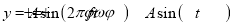(1)

where:

y = distance from the equilibrium position at time t

A = amplitude = maximum distance from equilibrium position

f = frequency = number of oscillations per second. An oscillation is one complete back-and-forth motion

ï· = angular frequency of the oscillation = 2ï°f

ïª = initial phase angle

T = The period of the oscillation,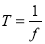.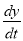= the velocity of the mass =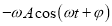.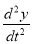= the acceleration of the mass =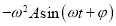.

## Theory

When a mass hangs from a (massless) spring and oscillates vertically, its period is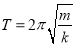where (2)

m = mass hanging from spring

k = spring constant (k = force/elongation)

Squaring both sides,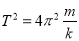If the spring’s mass is not negligible this becomes (for a uniform spring)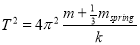,

which can be written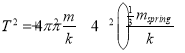.(3)

For a particular spring, this relation of period squared to mass can be written as a linear equation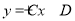where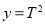and x = mass.

So a graph of T2 versus mass should be a straight line with

Slope =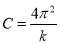(4)

Intercept =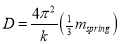(5)

### Pre lab assignment

1. Find the period and the frequency of an object that oscillates 30 times in 44 seconds.

2. In the sample graph, find the value of each of the following quantities; make sure you include proper units!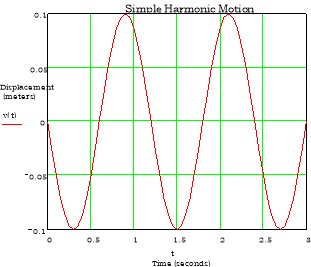amplitude

frequency

maximum velocity

maximum acceleration

initial phase angle (of position-time graph)

3.In the sample graph, at t ï€ 0.9 seconds, y = maximum. Explain why

v = 0

a = negative maximum

Also, at t ï€ 1.2 sec., y is at the midpoint of its oscillation. Explain why

v = negative maximum

a = 0

### Apparatus

Pasco 750 Interface

Motion sensor

Spring, 6 cm by 1.5 cm from Pasco track accessories

Large table clamp, right angle clamp, multi-position pendulum clamp and rods to hold spring and motion sensor (see Figure 1)

50 gram mass holder

50 grams of masses (1×10 gram and 2×20 gram masses)

Meterstick

Procedure and Analysis for the Simple Harmonic Motion Experiment

I.Set-up of computer and interface

1. Turn on the Pasco 750 interface first. Notice that the indicator light is on.
2. Turn on the computer and login.
3. Set up the equipment, as shown in Figure 1
4. Click on Data Studio, following separate Data Studio instructions.
5. Select Motion Sensor.
6. Double click on Motion to get to Sensor Properties.
7. Under Motion Sensor, increase trigger rate to 25 Hz.
8. Under Measurement, select position, and leave velocity and acceleration as selected.
9. Click and drag velocity from the Data Window, to the graph icon to create a velocity versus time graph.
10. Click and drag acceleration from the Data Window to the bottom of the velocity graph to create an acceleration graph below the velocity graph.
11. Click on the lock icon to keep the time axes of the plots locked together.
1. Set-up of equipment

Set-up a desk clamp and rods to hold the spring as in Figure 1. Hang the 50g weight holder from the spring, as shown in Figure 1.

 III.Measurement of elongation of spring versus applied force Hold the meterstick in a vertical position next to the weight holder, with the 100cm end touching the floor. Read the position of the bottom of the weight holder; record the total mass on the spring and the position of the mass into an excel spreadsheet. Add 10 grams to the holder and again read and record the mass and position into the excel spreadsheet. Repeat until the total hanging mass is 100 grams (the 50 gram holder plus 50 grams of weights.) This data will be graphed later to determine the spring constant of the spring. IV.Set-up of the motion sensor Use the desk clamp and rods to set up the motion sensor as in Figure 1. The motion sensor should be resting on the floor directly below the weight holder, and should be leveled so that its beam goes directly upward. Set the beam width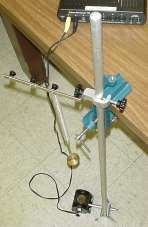Figure 1. Spring, hanging mass, motion sensor, and miscellaneous rods and clamps for the SHM experiment

switch on the sensor for narrow beam and connect the yellow plug to digital channel 1 of the Pasco interface, and the other plug to channel 2.

V.Recording of position-time data during oscillations

With just the 50 gram holder on the spring, raise or lower the rod holding the

spring until the bottom of the weight holder is about 40 centimeters above the motion sensor. This is done so that the distance from sensor to weight holder will never be less than about 30 centimeters during an oscillation. This is to insure that the motion sensor accurately measures the distance.

Start the weight holder oscillating vertically, about 5 centimeters above and below the equilibrium position. Click on START to begin recording. After a minimum of 5 oscillations, click STOP.

VI.Determining the oscillation frequency by a sinusoidal fit

1. Click on Zoom to select the data to be fit. Go to Fit, and select Sine Series Fit. Fit the velocity data, and the acceleration data separately. The data points should form a smooth sine curve. If they don’t, delete the data and record data again. To delete the data, click on run#1 in the experiment set-up window, hit delete, and click on OK.
2. The fitted curve should match the data.
3. Into a second excel spreadsheet, record the mass on the spring, the amplitude of the velocity, of the acceleration, the frequency of the velocity, and the acceleration.
4. Print out a few representative graphs to be included with your laboratory report.

5. Increase the hanging mass to 60g (total) and again adjust the spring support so that the mass hanger is about 50 cm above the motion sensor. Repeat V and VI.

6. Repeat the above steps for a total mass of 70, 80, 90, and 100 grams

7. Finally, disassemble the apparatus and measure the mass of the spring on a balance.

VII.Calculations

EQUATIONS:-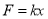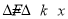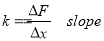09/20/161

View all

## DMCA / Removal Request

If you are the original writer of this essay and no longer wish to have your work published on UKEssays.com then please:

Related Services

Prices from

KD47

Approximate costs for:

• 1000 words
• 7 day deliveryHumanity University

Dedicated to your worth and value as a human being!

Related Lectures